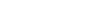Hello,

I just purchased two of the ADC121C_ACS714_1Channel_Dc_Current_Monitoring_Sensor from NCD. I’m using Raspberry Pi and this library to read the current.

However, I’m getting 2.054A when even there is no current to read. I see the problem on both sensors so I don’t think its a bad component. I read Register 0, which according to the spec holds the latest ADC conversion value, and I get 0x806. Shouldn’t this register be zero when there is no circuit being sensed?

Is there any calibration I need to do beforehand?

Hi,
This is a bi directional current sensor and when no current is flowing it will read 2.048V.
The reason behind is that while current flow from one directional to another the value will go from 2.048V to 5V and if the current directional changes the values will go from 2.048V to 0.

Thanks

Ok. How do you then convert the value from Voltage to Current? For example, if I’m getting 2.078V reading. How do I convert that to current?

Amps = (Volt * 1000 - 2500)/185

in your case if the volt is 2.078V
Amp = (2.078 * 1000 - 2500)/185
Amp = 2.28A

Thanks

The equation doesnt make sense to me.

If there is no current flowing, the value on register 0 will be 0x800 which translates to 2.048V. Is that right?

Then, to convert this to Amperage, we use your equation: Amps = (Volt*1000-2500)/185

If I plug in 2.048V into that equation, I get a non zero value (-2.44A) even though there is no current flowing. Is there something else I’m missing?

Amps = (2.048*1000-2500)/185 = -2.44A

the equation is assuming that your vref/vdd is 5V.

if no current is flowing and your Vout = 2.048 then then 2500 value will be replaced by 2.48

Thanks

Are you saying that, if there is no current, the equation becomes `Amps = (Volt * 1000 - 2.48)/185` ?

That doesn’t look right. Did you mean `Amps = (Volt * 1000 - 2048)/185`?

Is there a python script or example that I can use to automate the calculation?